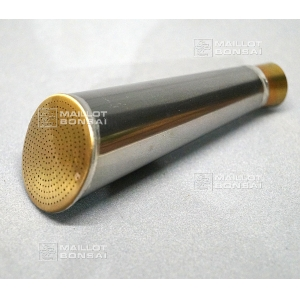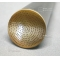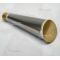##### The Japanese Bonsai specialist
Direct order Contact Help / Services Newsletter# Noozle for watering inox can 4/6 liter ø 50 mm

› Bonsai tools › Watering equipment › Copper watering canref. : 10101

25,00

Available quantity : 5Order

###### Description

For watering-can 4 and 6 liters made of steel. Dimension of the throw 50 mm. Diameter interior mouth: 14 millimeters. Outside 16 mm. Body steel and grid made of yellow copper. Very thin throw. "The technique of the laser permits a regularity and a precision of drilling that give to this new nozzle a throw and a sweetness of watering more adapted to the bonsaïs ".

#watering 6.8 #copper 3.6 #throw 3.5 #noozle 2.6 #liter 2.5 #steel 2.5 #made 2.4 #inox 2.4 #millimeters 2.1 #regularity 2

Formule
(( ROUND((CHAR_LENGTH(b.article_nom)-CHAR_LENGTH(REPLACE(b.article_nom, 'watering', '')))/LENGTH('watering')) + ROUND((CHAR_LENGTH(b.article_description)-CHAR_LENGTH(REPLACE(b.article_description, 'watering', '')))/LENGTH('watering')) ) * 4.8) + (( ROUND((CHAR_LENGTH(b.article_nom)-CHAR_LENGTH(REPLACE(b.article_nom, 'throw', '')))/LENGTH('throw')) + ROUND((CHAR_LENGTH(b.article_description)-CHAR_LENGTH(REPLACE(b.article_description, 'throw', '')))/LENGTH('throw')) ) * 3.5) + (( ROUND((CHAR_LENGTH(b.article_nom)-CHAR_LENGTH(REPLACE(b.article_nom, 'noozle', '')))/LENGTH('noozle')) + ROUND((CHAR_LENGTH(b.article_description)-CHAR_LENGTH(REPLACE(b.article_description, 'noozle', '')))/LENGTH('noozle')) ) * 2.6) + (( ROUND((CHAR_LENGTH(b.article_nom)-CHAR_LENGTH(REPLACE(b.article_nom, 'liter', '')))/LENGTH('liter')) + ROUND((CHAR_LENGTH(b.article_description)-CHAR_LENGTH(REPLACE(b.article_description, 'liter', '')))/LENGTH('liter')) ) * 2.5) + (( ROUND((CHAR_LENGTH(b.article_nom)-CHAR_LENGTH(REPLACE(b.article_nom, 'steel', '')))/LENGTH('steel')) + ROUND((CHAR_LENGTH(b.article_description)-CHAR_LENGTH(REPLACE(b.article_description, 'steel', '')))/LENGTH('steel')) ) * 2.5) + (( ROUND((CHAR_LENGTH(b.article_nom)-CHAR_LENGTH(REPLACE(b.article_nom, 'inox', '')))/LENGTH('inox')) + ROUND((CHAR_LENGTH(b.article_description)-CHAR_LENGTH(REPLACE(b.article_description, 'inox', '')))/LENGTH('inox')) ) * 2.4) + (( ROUND((CHAR_LENGTH(b.article_nom)-CHAR_LENGTH(REPLACE(b.article_nom, 'made', '')))/LENGTH('made')) + ROUND((CHAR_LENGTH(b.article_description)-CHAR_LENGTH(REPLACE(b.article_description, 'made', '')))/LENGTH('made')) ) * 2.4) + (( ROUND((CHAR_LENGTH(b.article_nom)-CHAR_LENGTH(REPLACE(b.article_nom, 'millimeters', '')))/LENGTH('millimeters')) + ROUND((CHAR_LENGTH(b.article_description)-CHAR_LENGTH(REPLACE(b.article_description, 'millimeters', '')))/LENGTH('millimeters')) ) * 2.1) + (( ROUND((CHAR_LENGTH(b.article_nom)-CHAR_LENGTH(REPLACE(b.article_nom, 'regularity', '')))/LENGTH('regularity')) + ROUND((CHAR_LENGTH(b.article_description)-CHAR_LENGTH(REPLACE(b.article_description, 'regularity', '')))/LENGTH('regularity')) ) * 2) + (( ROUND((CHAR_LENGTH(b.article_nom)-CHAR_LENGTH(REPLACE(b.article_nom, 'dimension', '')))/LENGTH('dimension')) + ROUND((CHAR_LENGTH(b.article_description)-CHAR_LENGTH(REPLACE(b.article_description, 'dimension', '')))/LENGTH('dimension')) ) * 1.9)

## Secure payment## Delivery

Our logistic partners :04 74 55 23 48
Pépinière MAILLOT-BONSAÏ
Le Bois Frazy
01990 RELEVANT - FRANCE
on appointment